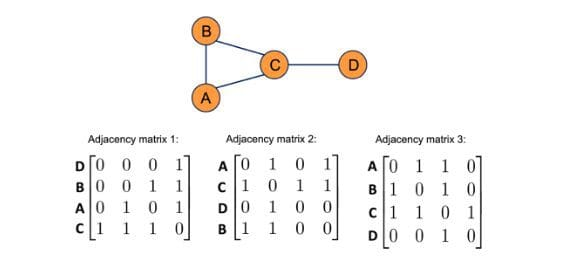# The motivation behind using graph convolutions

This article is an excerpt from the book Machine Learning with PyTorch and Scikit-Learn is the new book from the widely acclaimed and bestselling Python Machine Learning series, fully updated and expanded to cover PyTorch, transformers, graph neural networks, and best practices.

Graph Neural Networks (GNNs) has been an area of rapid development in recent years. According to the State of AI report from 2021, GNNs have evolved "from niche to one of the hottest fields of AI research."

This article is an excerpt from the book Machine Learning with PyTorch and Scikit-Learn is the new book from the widely acclaimed and bestselling Python Machine Learning series, fully updated and expanded to cover PyTorch, transformers, graph neural networks, and best practices.

In this section, we'll see why we want to use convolutions on graphs and discuss what attributes we want those convolutions to have.

In the context of images, we can think of convolution as the process of sliding a convolutional filter over an image, where, at each step, a weighted sum is computed between the filter and the receptive field (the part of the image it is currently on top of). The filter can be viewed as a detector for a specific feature. This approach to feature detection is well-suited for images for several reasons, for instance, the following priors we can place on image data:

1. Shift-invariance: We can still recognize a feature in an image regardless of where it is located (for example, after translation). A cat can be recognized as a cat whether it is in the top left, bottom right, or another part of an image.
2. Locality: Nearby pixels are closely related.
3. Hierarchy: Larger parts of an image can often be broken down into combinations of associated smaller parts. A cat has a head and legs; the head has eyes and a nose; the eyes have pupils and irises.

Another reason convolutions are well-suited for processing images is that the number of trainable parameters does not depend on the dimensionality of the input. You could train a series of 3×3 convolutional filters on, for example, a 256×256 or a 9×9 image. (However, if the same image is presented in different resolutions, the receptive fields and, therefore, the extracted features will differ. And for higher-resolution images, we may want to choose larger kernels or add additional layers to extract useful features effectively.)

Like images, graphs also have natural priors that justify a convolutional approach. Both kinds of data, images and graphs, share the locality prior. However, how we define locality differs. In images, the prior is on locality in 2D space, while with graphs, it is structural locality. Intuitively, this means that a node that is one edge away is more likely to be related than a node five edges away. For example, in a citation graph, a directly cited publication, which would be one edge away, is more likely to have similar subject matter than a publication with multiple degrees of separation.

A strict prior for graph data is permutation invariance, which means that the ordering of the nodes does not affect the output. This is illustrated in Figure 1, where changing the ordering of a graph's nodes does not change the graph's structure:Figure 1: Different adjacency matrices representing the same graph

Since the same graph can be represented by multiple adjacency matrices, as illustrated in Figure 1, consequently, any graph convolution needs to be permutation invariant.

A convolutional approach is also desirable for graphs because it can function with a fixed parameter set for graphs of different sizes. This property is arguably even more important for graphs than images. For instance, there are many image datasets with a fixed resolution where a fully connected approach (for example, using a multilayer perceptron) could be possible. In contrast, most graph datasets contain graphs of varying sizes. Learn more with Machine Learning with PyTorch and Scikit-Learn by Sebastian Raschka, Yuxi (Hayden) Liu, and Vahid Mirjalili.